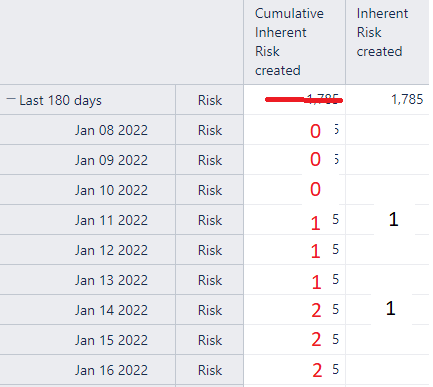# How to exclude first row in a Cumulative Sum with a dynamic time filter?

I want a cumulative sum of a custom measure of integer values that I have imported from Jira. This works in principle but the cumulative sum also sums the overall sum of my dynamic time filter - which creates an escalated starting point.

So how does one do a cumulative sum a measure based on a dynamic time filter (e.g. Last 180 days) without including the sum of the sums as the starting point:You can apply a date filter. Look at his example here: Date filters

Regards